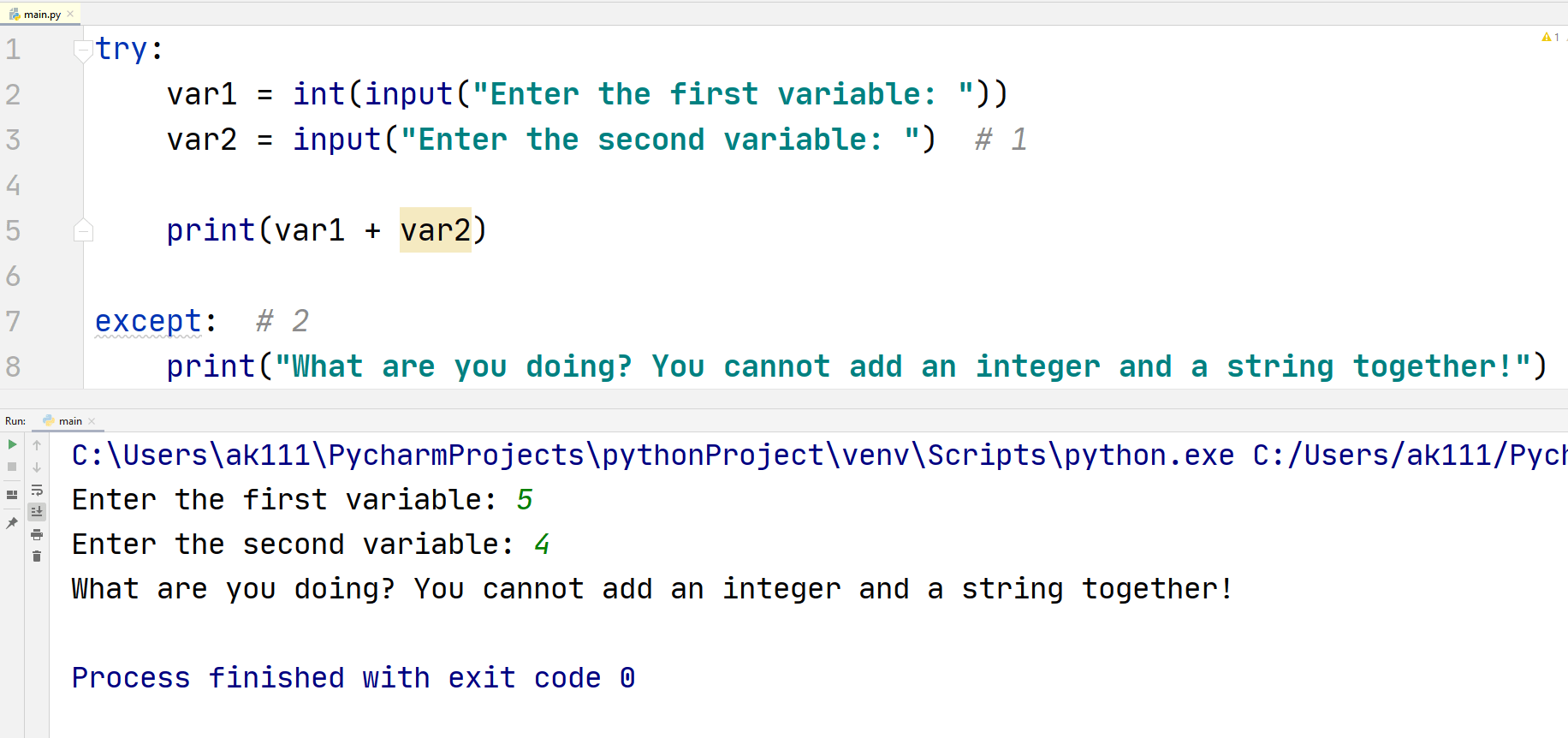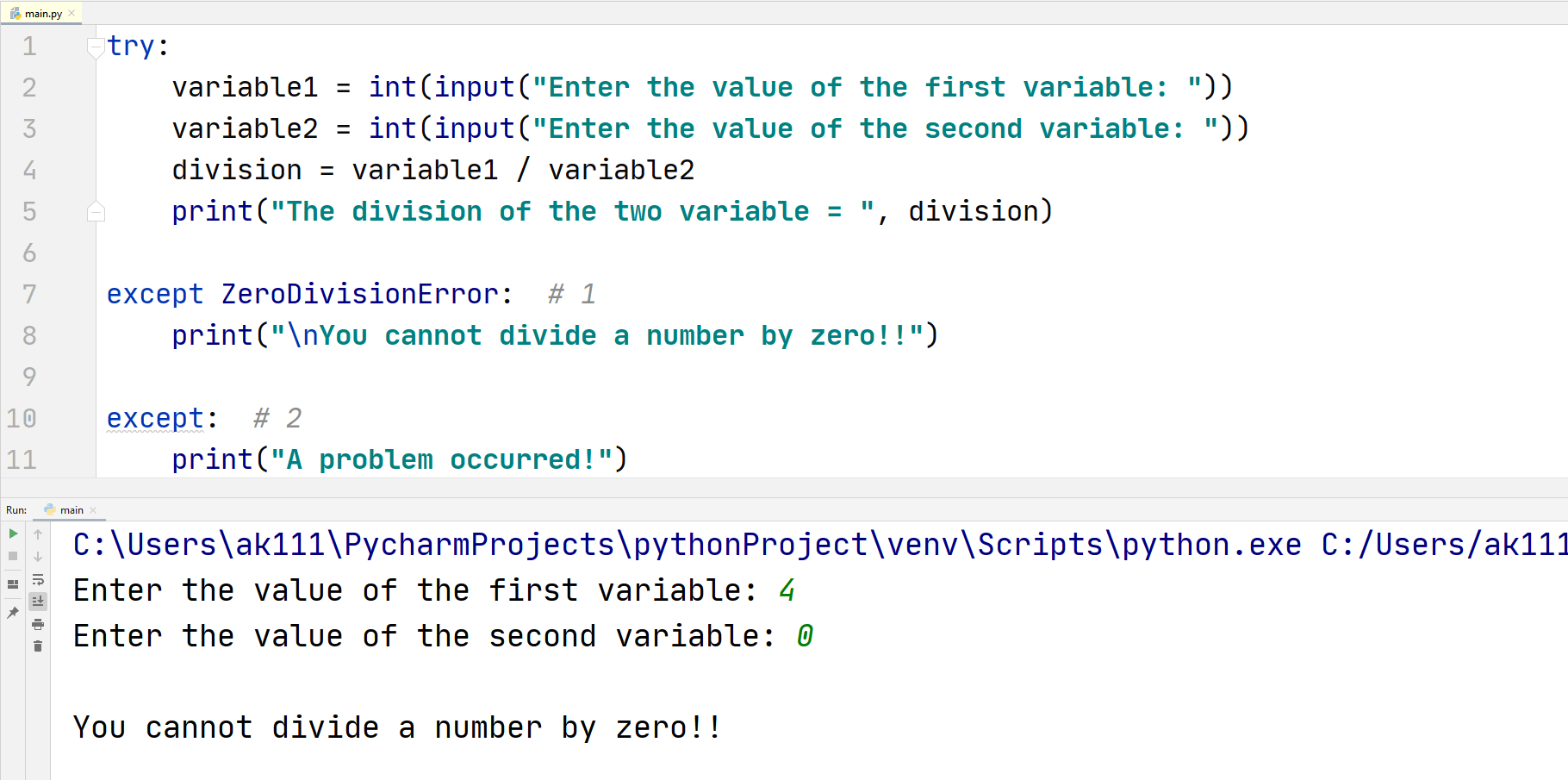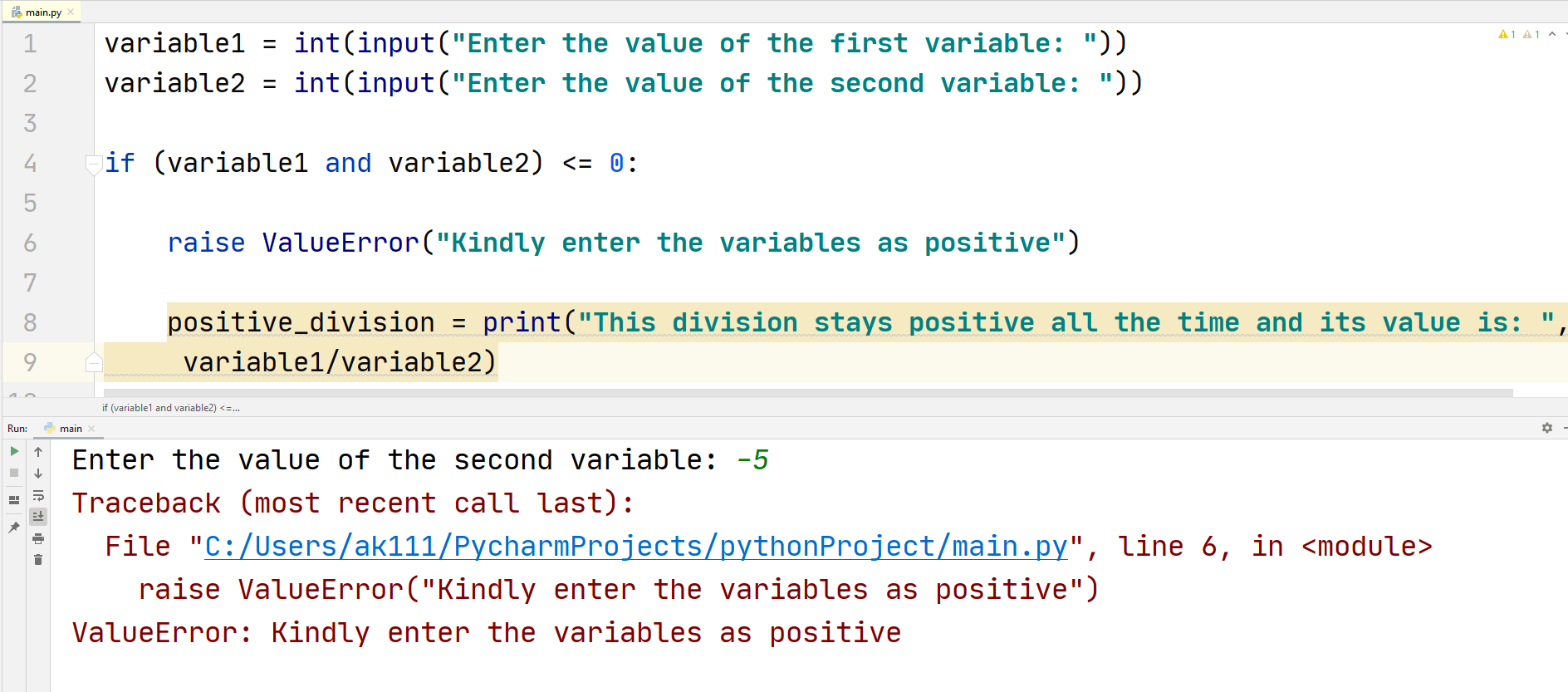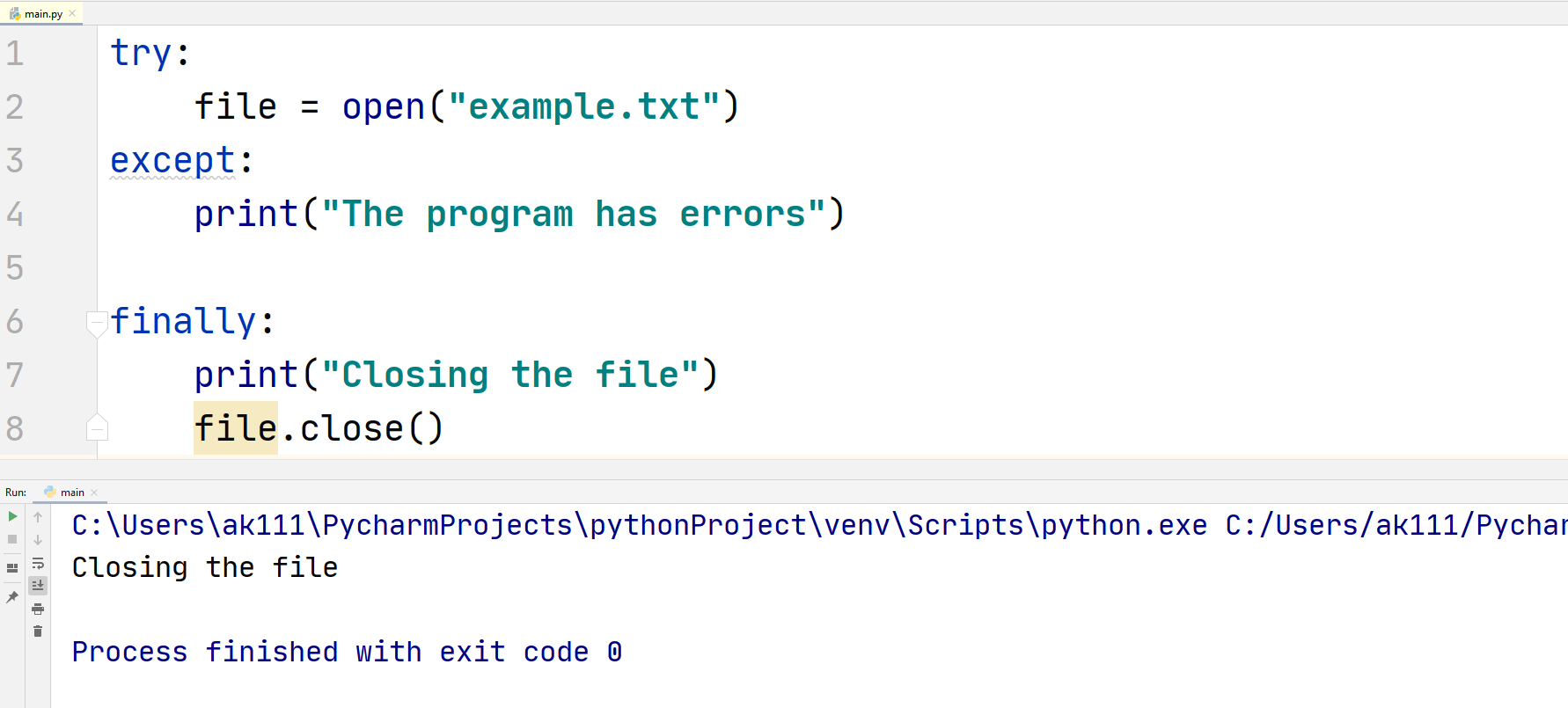# Python Try Except

## Python Try Except

Python has error messages to represent problems occurring in a code, two types of errors can occur in Python.

1. Syntax Error: This type of error usually occurs when Python does not understand a line of code that you have written. The syntax is specific to a programming language and should be correct and according to that programming language.

For example, if you forgot to add a bracket at the end of a print statement, Python will generate a syntax error.

2. Exception: This type of error occurs when Python successfully reads a line of code but while processing it, it has no idea of what to do with the line of code.

For example, when you try to add a string and an integer, Python will generate a TypeError exception that tells you that you cannot a string and an integer.

Basically, when an error occurs other than a syntax error, we call it an exception. Whenever an exception occurs, Python stops the code and generates an error message specific to the exception.

Python has many built-in exceptions, if we do not handle these exception messages, then the Python program will crash.

Therefore, most of the time it is necessary to handle these exceptions to prevent our program from crashing.

## Exception Handling in Python

Before handling an exception, you need to check your code for the exception. This is called catching an exception. This is done using the try block, and an except block that follows the try block is used to handle the exceptions.

Syntax:
try:
“code to be tested”
except:
“code to handle exception”

The syntax above can be understood better by taking an example of adding an integer and a string:

## ExampleHow is our code working?

1.  When you take an input, Python converts it in a string. So if you need to input an integer, you need to convert it back to the integer data type using the int function, something that we did not do in this line of the code.
2. When we tried to add those two variables, Python ran into a problem of adding two different data types together. So, when we used the except block, instead of giving us an error, Python printed what we wanted it to print.

## Multiple Exceptions

Let us take an example of a Situation:

• You have two integers and you want to divide variable1 by variable2, but variable2 is equal to zero. Everyone knows that you can’t divide a number by zero.
• In this situation, Python will generate a ZeroDivisionError but what if another exception can occur in the program, for example, you, by mistake, enter the value of one of the two variables equal to string.
• So, will Python still show the Exception error? The answer is yes, but this can easily be handled in the following way!

## ExampleCode Explanation:

1.  When we know what kind of exception can occur in the code, we can tell Python to follow a specific path for that exception. In this case when we divide by zero, Python goes to the except block of the ZeroDivisionError block.
2. For any other exception that can occur in Python except the ZeroDivisionError exception, Python will follow this code block.

This can also be referred to as catching specific exceptions in Python.

## Raise Exception

Exceptions in Python can also be generated manually using the raise keyword. Normally, Python generates exceptions when an error occurs while the code is running.

But if we want our code to only work on certain conditions, then it is a good idea to print out an error message to stop the code.

## Example## Ignore except Block

You cannot eradicate the except block completely from your code unless you have a finally block (discussed later in the article), but if you wish to do nothing if an exception occurs, the pass statement can be written in the except block to make the code run without an error.

## ExampleTips:
This is not recommended as this does not give you a hint of a problem occurring in the code. Even if there is a problem in the code, output will show nothing.

This is useful when you already know that your code is working and providing results but a specific IDE requires your code to meet certain requirements, and you do not want to work according to that IDE.

It is a normal situation that can happen in competitive programming.

## else Block

The else block can be added after the except block to execute if Python does not find exception errors in the code.

This is quite useful when you want to check for a block of code and then do something if that block of code runs without any error.

Consider this for an example:

## ExampleThis solves the problem of entering the wrong value of a number, if the user enters the value of the first variable and it turns out to be wrong, the code will go to the except block but if the value is right for the program, then the code will ask to input the second number.

### finally Block

The try block in the code can accept a finally block that would get executed every time you run your program. It does not care if your code has errors or the code runs smoothly.

You may wonder what is the use of this block anyways? The answer is that it is usually used to close opened files or objects.

## ExampleAs you can see that our program opened the file and then closed it. This is very useful when you have opened the file in the try block, but it might give an error. By using the finally block, you can close that file and move on for the rest of code even if the file generated an error or exception.